Next: Implementation Up: Number of roots of Previous: Number of roots of   Contents

Mathematical background

We use the half angle tangent substitution. If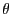is the unknown we define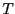as: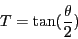Then we have: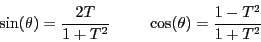Note that the change of variable is not valid if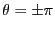. In that case it will be preferable to define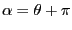and to transform the initial into an equation in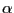. Then the change of variable may be applied.

Using the above relation any trigonometric equation can be transformed into a polynomial equation which is analyzed using the tools of chapter 5.

It remains to define an interval for angles that we will denote an angle interval. The element of an angle interval is usually defined between 0 and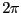(although in most of the following procedures any value can be used when not specified: internally the element of the angle interval are converted into value within this range). A difference between numbers interval (INTERVAL) and angle interval is that the lower bound of an angle interval may be larger than the upper bound. Indeed the order in an angle interval is important: for example the angle intervals [0,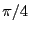] and [,0] are not the same.Next: Implementation Up: Number of roots of Previous: Number of roots of   Contents
Jean-Pierre Merlet 2012-12-20